# AP Calculus BC : Continuity in Terms of Limits

## Example Questions

### Example Question #1 : Limits And Continuity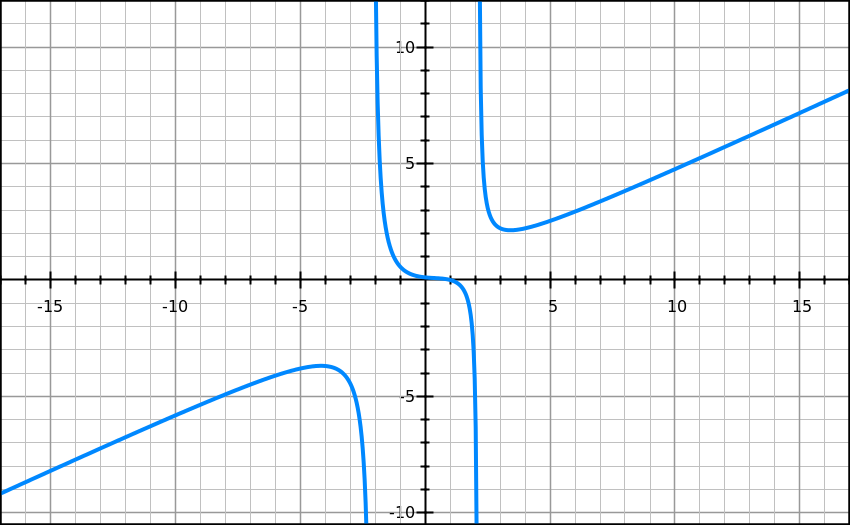The graph above is a sketch of the function. For what intervals iscontinuous?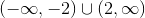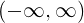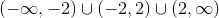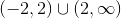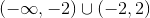Explanation:

For a function to be continuous at a point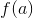must exist and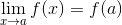This is true for all values ofexcept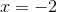and.

Therefore, the interval of continuity is.

### Example Question #1 : Limits And Continuity

Consider the piecewise function: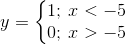What is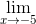?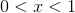Limit does not exist.Limit does not exist.

Explanation:

The piecewise function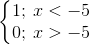indicates thatis one whenis less than five, and is zero if the variable is greater than five.  At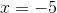, there is a hole at the end of the split.

The limit does not indicate whether we want to find the limit from the left or right, which means that it is necessary to check the limit from the left and right.  From the left to right, the limit approaches 1 asapproaches negative five.   From the right, the limit approaches zero asapproaches negative five.

Since the limits do not coincide, the limit does not exist for.

### Example Question #1 : Limits And Continuity

Consider the function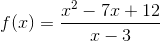.

I.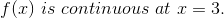II.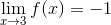III.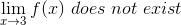I and II

II only

III only

I and III

I and II

Explanation:

For a function to be continuous at a particular point, the limit of the function at that point must be equal to the value of the function at that point.

First, notice that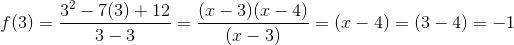This means that the function is continuous everywhere.

Next, we must compute the limit. Factor and simplify f(x) to help with the calculation of the limit.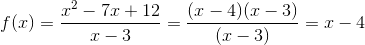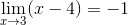Thus, the limit as x approaches three exists and is equal to, so I and II are true statements.

### All AP Calculus BC Resources